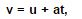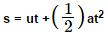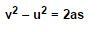# Acceleration due to gravity below and above the surface of earth

Any object falling free downward on earth has an acceleration of 9.8 m/s2. This a numerical value for the acceleration of a free-falling object is very important value therefore it is given a special name as the acceleration of gravity and physicists have a special symbol to denote it - the symbol is g. , the acceleration for any object moving under the sole influence of gravity. There are slight variations in this numerical value in the second decimal place that is dependent primarily upon on altitude. Some times we use the approximated value of 10 in calculations in order to reduce the complexity of the many mathematical tasks so that we will perform with this number. The value 10 is taken so that we will be able to better focus on the conceptual nature of physics without too much of a sacrifice in numerical accuracy.

Acceleration due to gravity is the acceleration gained by an object due to gravitational force.

Its SI unit is m/s2. It has both magnitude and direction, therefore it’s a vector quantity. The Acceleration due to gravity formula is as follows:Where

• V = final Velocity
• u=initial velocity
• a = acceleration
• t= time taken
• ### Factors affecting acceleration due to gravity

The shape and rotational motion of the earth

Altitude above the earth's surface and

Depth below the earth's surface.